Next: Conclusions Up: tr04mw1 Previous: Results

# Discussion

It is worth considering the different sources of individual class broadening (variance) in the image intensity histogram. One source is intrinsic within-class variation in the underlying signal, for example, FMRI, where the activation strength does vary within an "activated" cluster. Secondly, broadening is caused by image noise, both high frequency noise and also, for example, in the case of structural brain images, low frequency noise or "bias field". A third cause is limitations of the model, for example, in the case of the models described in this paper, partial volume effect (PVE), where a voxel in reality contains a mixture of different classes. In Santago and Gage (1995); Ruan et al. (2000) spatial neighbourhood information is used to aid classification into pure voxels and PVE voxels. A very similar approach to PVE models is that of fuzzy mixture segmentation (Caillol et al., 1997). The approach in our paper, using the language of Caillol et al. (1997) is a hard segmentation'' in that the intention is to find posterior pdfs on discrete classification labels. The fuzzy approach of Caillol et al. (1997), like PVE modelling, consider voxels as being mixtures of classes rather than discretely belonging to one class or another. In PVE models (or fuzzy mixture models), the resulting models have weights vectors at each voxel to specify the mixture of the different classes. However, this should not be confused with the weights vectors used in this paper, which by virtue of the logistic transform are not producing mixtures of classes at a voxel but instead an approximation to discrete labels (to perform a hard segmentation).

Nevertheless, it might be of interest to extend the models described in this paper to include PVE estimation. Note, however, that whilst this makes sense conceptually for situations where the original idealised class distribution is a delta-function (e.g., structural image segmentation), it is more complex for situations where real class intensities vary within-class (e.g., FMRI activation map classification). This is because there is now an ambiguity between varying PVE fractions and real within-class signal variations; in fact, when considering the underlying causes of variation in activation intensity in FMRI, the two issues (varying activation strength and PVE) may well be physically/physiologically the same thing, in which case it may make no sense to attempt separate PVE (or fuzzy) modelling.

Commonly in FMRI, people use null hypothesis testing to label voxels, or clusters of voxels, as being active'' if they reject the null hypothesis at a given false positive rate (FPR) (Friston et al., 1995). This depends on knowing the null distribution (or non-activation distribution) for relevant statistics under the null hypothesis.

In contrast, spatial mixture modelling provides us with a way of estimating the activating'' and non-activating'' distributions from the data itself. One advantage of estimating the non-activating'' distribution (in particular) from the data is that it allows some adjustment for modelling assumption violations. An example of the value of explicit separate models for background and activation distributions is that common problems in time-series modelling (such as imperfect autocorrelation modelling or the presence of structured noise) can cause the "null" distribution to be both shifted and scaled away from its assumed form, invalidating null-hypothesis testing. However, it is worth noting that the limitation of spatial mixture modelling for FMRI (or any other application) inference is the validity of the distributional assumptions. Whilst the adaptivity of the parameters of the Gaussian distribution we use to model the non-activating'' class can protect against small modelling assumption violations, this will not protect us against all modelling assumption violations.

There is also a well-known problem in null hypothesis testing of FMRI. The problem is that if enough observations are made, then every voxel in the brain will reject the null hypothesis (Friston et al., 2002). This is because in practice no voxels will show completely no reponse to the stimulus, if only due to modelling inadequacies such as unmodelled stimulus correlated motion or the point spread function of the scanner. By doing mixture modelling we can overcome this by instead of asking the question Is the activation zero or not?'', we ask the question Is the activation bigger than the overall background level of activation''?''.

There is also the question of inference flexiblity. Because we have both the activating'' and non-activating'' distributions we can calculate the probability of a voxel being activating'' and the probability of a voxel being non-activating''. This provides us with far more inference flexiblity compared with null hypothesis testing. We can still look to control the FPR by thresholding using the probability of a voxel being non-activating''. But now we could also look to control the true postive rate (TPR) by thresholding using the probability of a voxel being activating''. Controlling the TPR may be of real importance when using FMRI for pre-surgery planning. Usually, however, we would use a third option of comparing a voxel's probabilities of being in each of the classes. In this case there is a natural choice of threshold of assigning a voxel to the class with highest probability of membership.

There are other limitations of current FMRI null hypothesis techniques. A major issue is the incorporation of spatial information. The most popular method for this is via Gaussian Random Field Theory (Friston et al., 1994; Worsley et al., 1992). Gaussian Random Field Theory provides null distributions for clusters of voxels in SPMs. The problem is that to form clusters an arbitrary threshold has to be chosen (there is no objective way of setting this threshold, and its choice has a huge impact on the final thresholded results). Also, to meet the assumptions of Gaussian Random Field Theory, preprocessing spatial smoothing needs to be performed, the amount of which is another arbitrary choice. This is in stark contrast to the approach described in this paper for which there are no parameter choices to be made, apart from the final inference threshold.

In Fernandez and Green (2002) mixture models are used for disease mapping, in which the data is a count (the number of observed cases of disease) in each region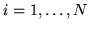that constitutes a geographical partition of the area of interest. Compellingly, they allow for the adaptive determination of the number of mixtures in the data via the use of reversible jumps MCMC sampling (Green, 1995). However, in tissue-type segmentation and FMRI activation classification the number of classes is normally known. Indeed, even in the absence of an assumed class, classifications were shown to be forced to zero using our method on artificial data when no activation or deactivation is supported by the data.

Fernandez and Green (2002) use a model related to our discrete labels non-spatial mixture model with class proportions (equation 5). However, they actually integrate out the discrete labels as they are not interested in classification. Instead, they are effectively looking to fit a different mixture at each voxel. This would mean allowing the class proportion parameters,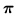, and the distribution parameters,, to vary locally. However, they restrict themselves to only allowing the class proportion parameters,, to vary locally (i.e.). Sanjay-Gopal and Hebert (1998) and Marroquin et al. (2003) use similar models, but they have to hand-tune the parameter controlling the amount of spatial regularisation of these class proportion parameters. In contrast, Fernandez and Green (2002) adaptively determine the amount of spatial regularisation. To spatially regularise using a Gaussian MRF they need to map the local class proportion parameters(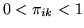,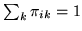) to random variables which are uniform from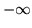to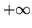. Hence, as we do in this paper, they also use the logistic transform. However, to maintain the interpretation of class proportion parameters they need to use a largein equation 12 (they require uniformity between 0 and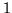for, whereas we required delta functions at 0 andfor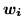, hence we used a small value of).

An interesting extension of the work in this paper would be to use Fernandez and Green (2002)'s non-stationary mixtures, whilst maintaining the spatially regularised classification of this paper. Non-stationary mixtures would allow local adaptation to spatial variations in the class distributions of intensity values such as can be observed particularly in structural brain MR images. The approach could have a non-stationary locally varying mixture (spatially regularisedand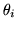) with spatially regularised classification,, with the strengths of spatial regularisation determined adaptively and separately.

On a 2GHz Intel PC the technique takes approximately 30mins on a full volume. Future work needs to be done on alternative inference techniques to MCMC, which could speed up the inference on the model specified in this paper considerably. Broadly, there are two possiblities: (1) a point estimation (maximum a posterior) approach such as ICM or simulated annealing, (2) full posterior approximation approaches such as Laplace or Variational Bayes.Next: Conclusions Up: tr04mw1 Previous: Results